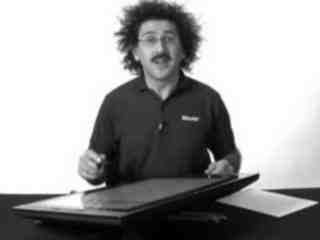Raffi Hovasapian

Further Examples with Extrema

Slide Duration:

Section 1: Vectors
Points & Vectors

28m 23s

Intro
0:00
Points and Vectors
1:02
A Point in a Plane
1:03
A Point in Space
3:14
Notation for a Space of a Given Space
6:34
Introduction to Vectors
9:51
14:51
Example 1
16:52
18:24
Example 2
21:01
Two More Properties of Vector Addition
24:16
Multiplication of a Vector by a Constant
25:27
Scalar Product & Norm

30m 25s

Intro
0:00
Scalar Product and Norm
1:05
Introduction to Scalar Product
1:06
Example 1
3:21
Properties of Scalar Product
6:14
Definition: Orthogonal
11:41
Example 2: Orthogonal
14:19
Definition: Norm of a Vector
15:30
Example 3
19:37
Distance Between Two Vectors
22:05
Example 4
27:19
More on Vectors & Norms

38m 18s

Intro
0:00
More on Vectors and Norms
0:38
Open Disc
0:39
Close Disc
3:14
Open Ball, Closed Ball, and the Sphere
5:22
Property and Definition of Unit Vector
7:16
Example 1
14:04
Three Special Unit Vectors
17:24
General Pythagorean Theorem
19:44
Projection
23:00
Example 2
28:35
Example 3
35:54
Inequalities & Parametric Lines

33m 19s

Intro
0:00
Inequalities and Parametric Lines
0:30
Starting Example
0:31
Theorem 1
5:10
Theorem 2
7:22
Definition 1: Parametric Equation of a Straight Line
10:16
Definition 2
17:38
Example 1
21:19
Example 2
25:20
Planes

29m 59s

Intro
0:00
Planes
0:18
Definition 1
0:19
Example 1
7:04
Example 2
12:45
General Definitions and Properties: 2 Vectors are Said to Be Paralleled If
14:50
Example 3
16:44
Example 4
20:17
More on Planes

34m 18s

Intro
0:00
More on Planes
0:25
Example 1
0:26
Distance From Some Point in Space to a Given Plane: Derivation
10:12
Final Formula for Distance
21:20
Example 2
23:09
Example 3: Part 1
26:56
Example 3: Part 2
31:46
Section 2: Differentiation of Vectors
Maps, Curves & Parameterizations

29m 48s

Intro
0:00
Maps, Curves and Parameterizations
1:10
Recall
1:11
Looking at y = x2 or f(x) = x2
2:23
Departure Space & Arrival Space
7:01
Looking at a 'Function' from ℝ to ℝ2
10:36
Example 1
14:50
Definition 1: Parameterized Curve
17:33
Example 2
21:56
Example 3
25:16
Differentiation of Vectors

39m 40s

Intro
0:00
Differentiation of Vectors
0:18
Example 1
0:19
Definition 1: Velocity of a Curve
1:45
Line Tangent to a Curve
6:10
Example 2
7:40
Definition 2: Speed of a Curve
12:18
Example 3
13:53
Definition 3: Acceleration Vector
16:37
Two Definitions for the Scalar Part of Acceleration
17:22
Rules for Differentiating Vectors: 1
19:52
Rules for Differentiating Vectors: 2
21:28
Rules for Differentiating Vectors: 3
22:03
Rules for Differentiating Vectors: 4
24:14
Example 4
26:57
Section 3: Functions of Several Variables
Functions of Several Variable

29m 31s

Intro
0:00
Length of a Curve in Space
0:25
Definition 1: Length of a Curve in Space
0:26
Extended Form
2:06
Example 1
3:40
Example 2
6:28
Functions of Several Variable
8:55
Functions of Several Variable
8:56
General Examples
11:11
Graph by Plotting
13:00
Example 1
16:31
Definition 1
18:33
Example 2
22:15
Equipotential Surfaces
25:27
Isothermal Surfaces
27:30
Partial Derivatives

23m 31s

Intro
0:00
Partial Derivatives
0:19
Example 1
0:20
Example 2
5:30
Example 3
7:48
Example 4
9:19
Definition 1
12:19
Example 5
14:24
Example 6
16:14
20:26
Higher and Mixed Partial Derivatives

30m 48s

Intro
0:00
Higher and Mixed Partial Derivatives
0:45
Definition 1: Open Set
0:46
Notation: Partial Derivatives
5:39
Example 1
12:00
Theorem 1
14:25
Now Consider a Function of Three Variables
16:50
Example 2
20:09
Caution
23:16
Example 3
25:42
Section 4: Chain Rule and The Gradient
The Chain Rule

28m 3s

Intro
0:00
The Chain Rule
0:45
Conceptual Example
0:46
Example 1
5:10
The Chain Rule
10:11
Example 2: Part 1
19:06
Example 2: Part 2 - Solving Directly
25:26
Tangent Plane

42m 25s

Intro
0:00
Tangent Plane
1:02
Tangent Plane Part 1
1:03
Tangent Plane Part 2
10:00
Tangent Plane Part 3
18:18
Tangent Plane Part 4
21:18
Definition 1: Tangent Plane to a Surface
27:46
Example 1: Find the Equation of the Plane Tangent to the Surface
31:18
Example 2: Find the Tangent Line to the Curve
36:54
Further Examples with Gradients & Tangents

47m 11s

Intro
0:00
Example 1: Parametric Equation for the Line Tangent to the Curve of Two Intersecting Surfaces
0:41
Part 1: Question
0:42
Part 2: When Two Surfaces in ℝ3 Intersect
4:31
Part 3: Diagrams
7:36
Part 4: Solution
12:10
Part 5: Diagram of Final Answer
23:52
Example 2: Gradients & Composite Functions
26:42
Part 1: Question
26:43
Part 2: Solution
29:21
Example 3: Cos of the Angle Between the Surfaces
39:20
Part 1: Question
39:21
Part 2: Definition of Angle Between Two Surfaces
41:04
Part 3: Solution
42:39
Directional Derivative

41m 22s

Intro
0:00
Directional Derivative
0:10
Rate of Change & Direction Overview
0:11
Rate of Change : Function of Two Variables
4:32
Directional Derivative
10:13
Example 1
18:26
Examining Gradient of f(p) ∙ A When A is a Unit Vector
25:30
Directional Derivative of f(p)
31:03
33:23
Example 2
34:53
A Unified View of Derivatives for Mappings

39m 41s

Intro
0:00
A Unified View of Derivatives for Mappings
1:29
Derivatives for Mappings
1:30
Example 1
5:46
Example 2
8:25
Example 3
12:08
Example 4
14:35
Derivative for Mappings of Composite Function
17:47
Example 5
22:15
Example 6
28:42
Section 5: Maxima and Minima
Maxima & Minima

36m 41s

Intro
0:00
Maxima and Minima
0:35
Definition 1: Critical Point
0:36
Example 1: Find the Critical Values
2:48
Definition 2: Local Max & Local Min
10:03
Theorem 1
14:10
Example 2: Local Max, Min, and Extreme
18:28
Definition 3: Boundary Point
27:00
Definition 4: Closed Set
29:50
Definition 5: Bounded Set
31:32
Theorem 2
33:34
Further Examples with Extrema

32m 48s

Intro
0:00
Further Example with Extrema
1:02
Example 1: Max and Min Values of f on the Square
1:03
Example 2: Find the Extreme for f(x,y) = x² + 2y² - x
10:44
Example 3: Max and Min Value of f(x,y) = (x²+ y²)⁻¹ in the Region (x -2)²+ y² ≤ 1
17:20
Lagrange Multipliers

32m 32s

Intro
0:00
Lagrange Multipliers
1:13
Theorem 1
1:14
Method
6:35
Example 1: Find the Largest and Smallest Values that f Achieves Subject to g
9:14
Example 2: Find the Max & Min Values of f(x,y)= 3x + 4y on the Circle x² + y² = 1
22:18
More Lagrange Multiplier Examples

27m 42s

Intro
0:00
Example 1: Find the Point on the Surface z² -xy = 1 Closet to the Origin
0:54
Part 1
0:55
Part 2
7:37
Part 3
10:44
Example 2: Find the Max & Min of f(x,y) = x² + 2y - x on the Closed Disc of Radius 1 Centered at the Origin
16:05
Part 1
16:06
Part 2
19:33
Part 3
23:17
Lagrange Multipliers, Continued

31m 47s

Intro
0:00
Lagrange Multipliers
0:42
First Example of Lesson 20
0:44
Let's Look at This Geometrically
3:12
Example 1: Lagrange Multiplier Problem with 2 Constraints
8:42
Part 1: Question
8:43
Part 2: What We Have to Solve
15:13
Part 3: Case 1
20:49
Part 4: Case 2
22:59
Part 5: Final Solution
25:45
Section 6: Line Integrals and Potential Functions
Line Integrals

36m 8s

Intro
0:00
Line Integrals
0:18
Introduction to Line Integrals
0:19
Definition 1: Vector Field
3:57
Example 1
5:46
Example 2: Gradient Operator & Vector Field
8:06
Example 3
12:19
Vector Field, Curve in Space & Line Integrals
14:07
Definition 2: F(C(t)) ∙ C'(t) is a Function of t
17:45
Example 4
18:10
Definition 3: Line Integrals
20:21
Example 5
25:00
Example 6
30:33
More on Line Integrals

28m 4s

Intro
0:00
More on Line Integrals
0:10
Line Integrals Notation
0:11
Curve Given in Non-parameterized Way: In General
4:34
Curve Given in Non-parameterized Way: For the Circle of Radius r
6:07
Curve Given in Non-parameterized Way: For a Straight Line Segment Between P & Q
6:32
The Integral is Independent of the Parameterization Chosen
7:17
Example 1: Find the Integral on the Ellipse Centered at the Origin
9:18
Example 2: Find the Integral of the Vector Field
16:26
Discussion of Result and Vector Field for Example 2
23:52
Graphical Example
26:03
Line Integrals, Part 3

29m 30s

Intro
0:00
Line Integrals
0:12
Piecewise Continuous Path
0:13
Closed Path
1:47
Example 1: Find the Integral
3:50
The Reverse Path
14:14
Theorem 1
16:18
Parameterization for the Reverse Path
17:24
Example 2
18:50
Line Integrals of Functions on ℝn
21:36
Example 3
24:20
Potential Functions

40m 19s

Intro
0:00
Potential Functions
0:08
Definition 1: Potential Functions
0:09
Definition 2: An Open Set S is Called Connected if…
5:52
Theorem 1
8:19
Existence of a Potential Function
11:04
Theorem 2
18:06
Example 1
22:18
Contrapositive and Positive Form of the Theorem
28:02
The Converse is Not Generally True
30:59
Our Theorem
32:55
Compare the n-th Term Test for Divergence of an Infinite Series
36:00
So for Our Theorem
38:16
Potential Functions, Continued

31m 45s

Intro
0:00
Potential Functions
0:52
Theorem 1
0:53
Example 1
4:00
Theorem in 3-Space
14:07
Example 2
17:53
Example 3
24:07
Potential Functions, Conclusion & Summary

28m 22s

Intro
0:00
Potential Functions
0:16
Theorem 1
0:17
In Other Words
3:25
Corollary
5:22
Example 1
7:45
Theorem 2
11:34
Summary on Potential Functions 1
15:32
Summary on Potential Functions 2
17:26
Summary on Potential Functions 3
18:43
Case 1
19:24
Case 2
20:48
Case 3
21:35
Example 2
23:59
Section 7: Double Integrals
Double Integrals

29m 46s

Intro
0:00
Double Integrals
0:52
Introduction to Double Integrals
0:53
Function with Two Variables
3:39
Example 1: Find the Integral of xy³ over the Region x ϵ[1,2] & y ϵ[4,6]
9:42
Example 2: f(x,y) = x²y & R be the Region Such That x ϵ[2,3] & x² ≤ y ≤ x³
15:07
Example 3: f(x,y) = 4xy over the Region Bounded by y= 0, y= x, and y= -x+3
19:20
Polar Coordinates

36m 17s

Intro
0:00
Polar Coordinates
0:50
Polar Coordinates
0:51
Example 1: Let (x,y) = (6,√6), Convert to Polar Coordinates
3:24
Example 2: Express the Circle (x-2)² + y² = 4 in Polar Form.
5:46
Graphing Function in Polar Form.
10:02
Converting a Region in the xy-plane to Polar Coordinates
14:14
Example 3: Find the Integral over the Region Bounded by the Semicircle
20:06
Example 4: Find the Integral over the Region
27:57
Example 5: Find the Integral of f(x,y) = x² over the Region Contained by r= 1 - cosθ
32:55
Green's Theorem

38m 1s

Intro
0:00
Green's Theorem
0:38
Introduction to Green's Theorem and Notations
0:39
Green's Theorem
3:17
Example 1: Find the Integral of the Vector Field around the Ellipse
8:30
Verifying Green's Theorem with Example 1
15:35
A More General Version of Green's Theorem
20:03
Example 2
22:59
Example 3
26:30
Example 4
32:05
Divergence & Curl of a Vector Field

37m 16s

Intro
0:00
Divergence & Curl of a Vector Field
0:18
Definitions: Divergence(F) & Curl(F)
0:19
Example 1: Evaluate Divergence(F) and Curl(F)
3:43
Properties of Divergence
9:24
Properties of Curl
12:24
Two Versions of Green's Theorem: Circulation - Curl
17:46
Two Versions of Green's Theorem: Flux Divergence
19:09
Circulation-Curl Part 1
20:08
Circulation-Curl Part 2
28:29
Example 2
32:06
Divergence & Curl, Continued

33m 7s

Intro
0:00
Divergence & Curl, Continued
0:24
Divergence Part 1
0:25
Divergence Part 2: Right Normal Vector and Left Normal Vector
5:28
Divergence Part 3
9:09
Divergence Part 4
13:51
Divergence Part 5
19:19
Example 1
23:40
Final Comments on Divergence & Curl

16m 49s

Intro
0:00
Final Comments on Divergence and Curl
0:37
Several Symbolic Representations for Green's Theorem
0:38
Circulation-Curl
9:44
Flux Divergence
11:02
Closing Comments on Divergence and Curl
15:04
Section 8: Triple Integrals
Triple Integrals

27m 24s

Intro
0:00
Triple Integrals
0:21
Example 1
2:01
Example 2
9:42
Example 3
15:25
Example 4
20:54
Cylindrical & Spherical Coordinates

35m 33s

Intro
0:00
Cylindrical and Spherical Coordinates
0:42
Cylindrical Coordinates
0:43
When Integrating Over a Region in 3-space, Upon Transformation the Triple Integral Becomes..
4:29
Example 1
6:27
The Cartesian Integral
15:00
Introduction to Spherical Coordinates
19:44
Reason It's Called Spherical Coordinates
22:49
Spherical Transformation
26:12
Example 2
29:23
Section 9: Surface Integrals and Stokes' Theorem
Parameterizing Surfaces & Cross Product

41m 29s

Intro
0:00
Parameterizing Surfaces
0:40
Describing a Line or a Curve Parametrically
0:41
Describing a Line or a Curve Parametrically: Example
1:52
Describing a Surface Parametrically
2:58
Describing a Surface Parametrically: Example
5:30
Recall: Parameterizations are not Unique
7:18
Example 1: Sphere of Radius R
8:22
Example 2: Another P for the Sphere of Radius R
10:52
This is True in General
13:35
Example 3: Paraboloid
15:05
Example 4: A Surface of Revolution around z-axis
18:10
Cross Product
23:15
Defining Cross Product
23:16
Example 5: Part 1
28:04
Example 5: Part 2 - Right Hand Rule
32:31
Example 6
37:20
Tangent Plane & Normal Vector to a Surface

37m 6s

Intro
0:00
Tangent Plane and Normal Vector to a Surface
0:35
Tangent Plane and Normal Vector to a Surface Part 1
0:36
Tangent Plane and Normal Vector to a Surface Part 2
5:22
Tangent Plane and Normal Vector to a Surface Part 3
13:42
Example 1: Question & Solution
17:59
Example 1: Illustrative Explanation of the Solution
28:37
Example 2: Question & Solution
30:55
Example 2: Illustrative Explanation of the Solution
35:10
Surface Area

32m 48s

Intro
0:00
Surface Area
0:27
Introduction to Surface Area
0:28
Given a Surface in 3-space and a Parameterization P
3:31
Defining Surface Area
7:46
Curve Length
10:52
Example 1: Find the Are of a Sphere of Radius R
15:03
Example 2: Find the Area of the Paraboloid z= x² + y² for 0 ≤ z ≤ 5
19:10
Example 2: Writing the Answer in Polar Coordinates
28:07
Surface Integrals

46m 52s

Intro
0:00
Surface Integrals
0:25
Introduction to Surface Integrals
0:26
General Integral for Surface Are of Any Parameterization
3:03
Integral of a Function Over a Surface
4:47
Example 1
9:53
Integral of a Vector Field Over a Surface
17:20
Example 2
22:15
Side Note: Be Very Careful
28:58
Example 3
30:42
Summary
43:57
Divergence & Curl in 3-Space

23m 40s

Intro
0:00
Divergence and Curl in 3-Space
0:26
Introduction to Divergence and Curl in 3-Space
0:27
Define: Divergence of F
2:50
Define: Curl of F
4:12
The Del Operator
6:25
Symbolically: Div(F)
9:03
Symbolically: Curl(F)
10:50
Example 1
14:07
Example 2
18:01
Divergence Theorem in 3-Space

34m 12s

Intro
0:00
Divergence Theorem in 3-Space
0:36
Green's Flux-Divergence
0:37
Divergence Theorem in 3-Space
3:34
Note: Closed Surface
6:43
Figure: Paraboloid
8:44
Example 1
12:13
Example 2
18:50
Recap for Surfaces: Introduction
27:50
Recap for Surfaces: Surface Area
29:16
Recap for Surfaces: Surface Integral of a Function
29:50
Recap for Surfaces: Surface Integral of a Vector Field
30:39
Recap for Surfaces: Divergence Theorem
32:32
Stokes' Theorem, Part 1

22m 1s

Intro
0:00
Stokes' Theorem
0:25
Recall Circulation-Curl Version of Green's Theorem
0:26
Constructing a Surface in 3-Space
2:26
Stokes' Theorem
5:34
Note on Curve and Vector Field in 3-Space
9:50
Example 1: Find the Circulation of F around the Curve
12:40
Part 1: Question
12:48
Part 2: Drawing the Figure
13:56
Part 3: Solution
16:08
Stokes' Theorem, Part 2

20m 32s

Intro
0:00
Example 1: Calculate the Boundary of the Surface and the Circulation of F around this Boundary
0:30
Part 1: Question
0:31
Part 2: Drawing the Figure
2:02
Part 3: Solution
5:24
Example 2: Calculate the Boundary of the Surface and the Circulation of F around this Boundary
13:11
Part 1: Question
13:12
Part 2: Solution
13:56
Bookmark & Share Embed

## Copy & Paste this embed code into your website’s HTML

Please ensure that your website editor is in text mode when you paste the code.
(In Wordpress, the mode button is on the top right corner.)
×
• - Allow users to view the embedded video in full-size.
Since this lesson is not free, only the preview will appear on your website.

• ## Related Books1 answerLast reply by: Professor HovasapianTue Jun 3, 2014 7:19 PMPost by Juan Castro on May 22, 2014Raffi,This lecture could have been hard but you made it seemingly easy. Great teaching style. Thanks!1 answerLast reply by: Professor HovasapianFri Apr 12, 2013 12:32 AMPost by Jawad Hassan on April 11, 2013the last example was so big, do you think this is normal problems in a exam? or is too long for a exam problem?

### Further Examples with Extrema

Find the maximum and minimum values of f(x,y) = 2x − 2y2 on the region D = { (x,y)| − 1x ≤ 1, − 1 ≤ y ≤ 1} .
• The region D is closed and bounded and f is continuous on D, so there exists a maximum and minimum value on D. We first look for critical points on that region.
• So [df/dx] = 0 implies 2 = 0 which is not true. Also [df/dy] = − 4y = 0 implies y = 0. Then our critical points occurt anywhere at f(x,0) = 2x, that is, along our x - axis. Note that this is an increasing function on the xz - plane.
•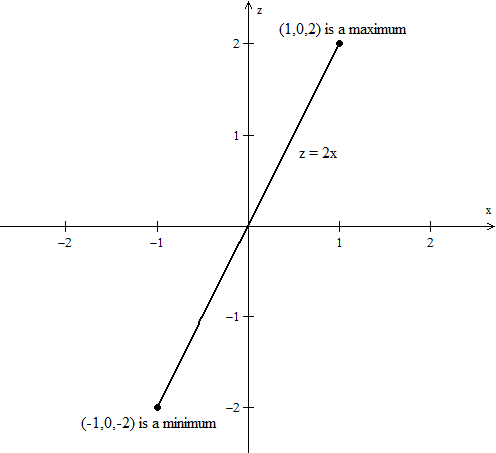• We now have f( − 1,0) and f(1,0) as extrema. Since f is increasing we must check the endpoints of our region D for extrema as well.
•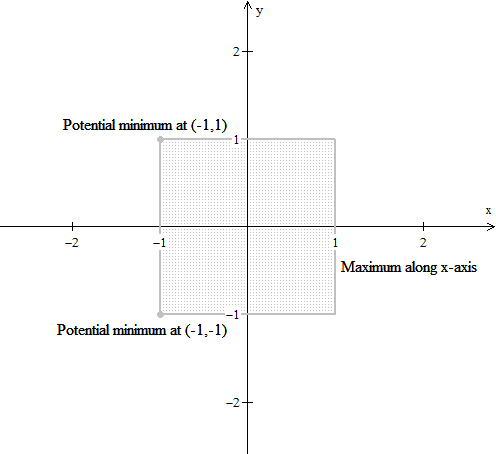• Now f( − 1,1) = − 4 and f( − 1, − 1) = − 4. We see that both of these values are less than f( − 1,0) = − 2.
Thus our maximum point is (1,0,2), our minimum points are ( − 1,1, − 4) and ( − 1, − 1, − 4)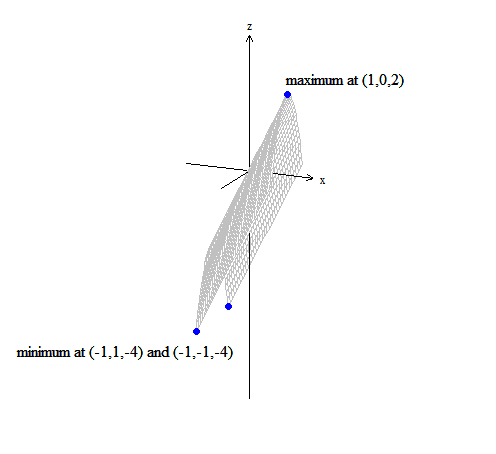Find the maximum and minimum values of f(x,y) = √x − y + xy on the region D = { (x,y)|0 ≤ x ≤ 2,0 ≤ y ≤ 1} .
• The region D is closed and bounded and f is continuous on D, so there exists a maximum and minimum value on D. We first look for critical points on that region.
• So [df/dx] = [1/(2√x )] + y also [df/dy] = − 1 + x = 0 implies x = 1. Hence [1/(2√1 )] + y = 0 implies y = − [1/2] and so our critical value is at ( 1, − [1/2] ). Note that ( 1, − [1/2] ) ∉ D.
•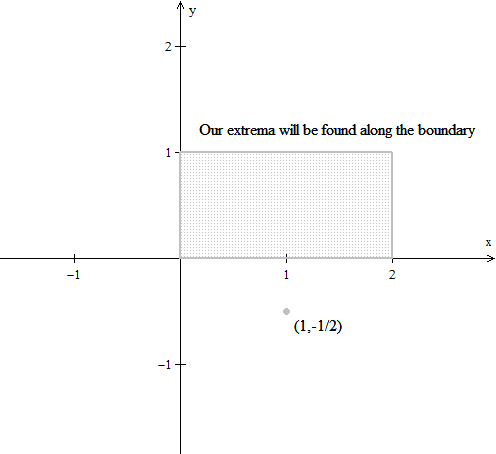• To check for extrema on our boundary we observe the four cases: f(0,y), f(2,y), f(x,0) and f(x,1).
• For f(0,y) we have z = − y whose maximum value on D occurs at y = 0 and minimum value occurs at y = 1. Our possible extrema are (0,0,0) and (0,1, − 1).
• For f(2,y) we have z = √2 − y + 2y = √2 + y an increasing function on D. The minimum value occurs at y = 0 and maximum value at y = 1. Our possible extrema are (2,0,√2 ) and (2,1,√2 + 1).
• For f(x,0) we have z = √x which is an increasing function. On D the minimum value occurst at x = 0 and the maximum value at x = 2. Our possible extrema are (0,0,0) and (2,0,√2 ).
• For f(x,1) we have z = √x − 1 + x. Note that √x − 1 and x are increasing functions and so z = √x − 1 + x is also increasing. Our possible extrema are at (0,1, − 1) and (2,1,√2 + 1).
Note that our extrema occur at the corners of our boundary. The minimum value is (0,1, − 1) and our maximum value is (2,1,√2 + 1).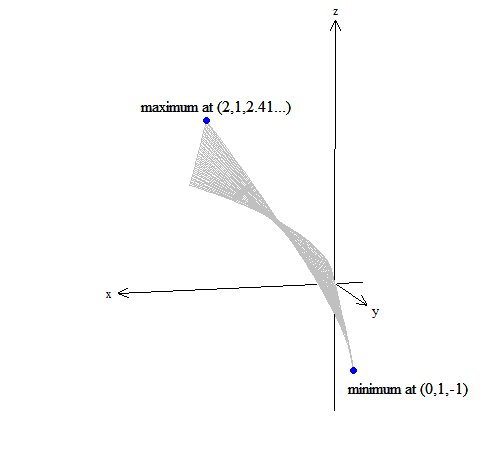Find the maximum and minimum values of f(x,y) = 3y2 − 4x2√y on the region D = { (x,y)| − 1 ≤ x ≤ 1,0 ≤ y ≤ 2} .
• The region D is closed and bounded and f is continuous on D, so there exists a maximum and minimum value on D. We first look for critical points on that region.
• So [df/dx] = 0 implies − 8x√y = 0 with solutions x = 0 or y = 0. Also [df/dy] = − 6y − [(2x2)/(√y )], if x = 0 we obtain y = 0. A critical value is (0,0,0).
• Since y0 we find that [df/dy] = − 6y − [(2x2)/(√y )] = 0 yields − 3 = ( [x/(y3 \mathord/ phantom 3 4/ 4)] )2 which is not possible.
• But recall that y = 0 is undefined at [df/dy], so our critical values are the points (x,0,0). Note that at x = 0 we have (0,0,0).
• Thus far our analysis has given us a possible minimums at (x,0,0).
• To check for extrema on our boundary we observe the four cases: f( − 1,y), f(1,y), f(x,0) and f(x,2).
• For f( − 1,y) we have z = 3y2 − 4√y which is a part of a parabola on D. We find the minimum value of the parabola (vertex) to be − 32 \mathord/ phantom 2 3 3, so our possible extrema becomes ( − 1,3 − 2 \mathord/ phantom 2 3 3, − 32 \mathord/ phantom 2 3 3 ).
•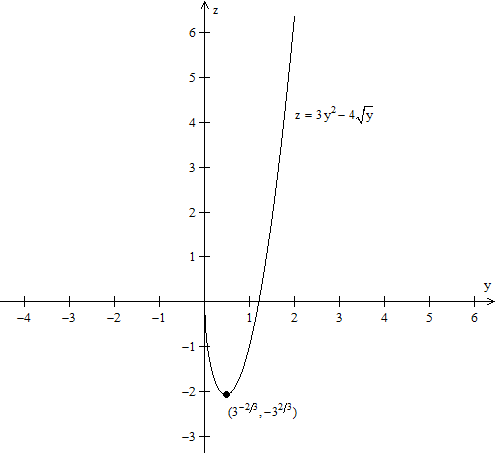• Similarly f(1,y) results in z = 3y2 − 4√y . We obtain the extrema ( 1,3 − 2 \mathord/ phantom 2 3 3, − 32 \mathord/ phantom 2 3 3 ).
• For f(x,0) we have already obserbed that our extrema is (x,0,0).
• For f(x,2) we have z = 12 − 4x2√2 which is a part of a parabola on D. We find the minimum value of the parabola (vertex) to be 12, a previously discovered possible extrema ( 0,2,12 ).
•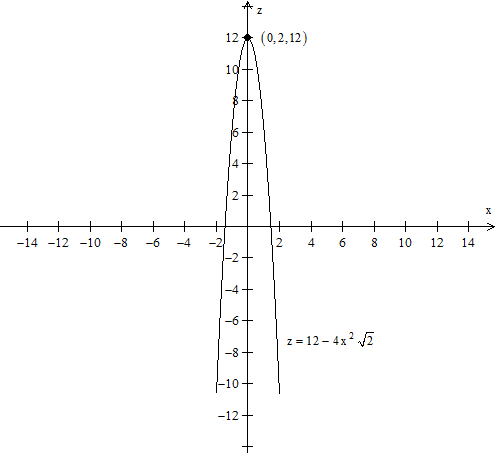Our possible extrema are then (x,0,0), (0,2,12), ( − 1,3 − 2 \mathord/ phantom 2 3 3, − 32 \mathord/ phantom 2 3 3 ), ( 1,3 − 2 \mathord/ phantom 2 3 3, − 32 \mathord/ phantom 2 3 3 ). Hence our minimum values are ( − 1,3 − 2 \mathord/ phantom 2 3 3, − 32 \mathord/ phantom 2 3 3 ) and ( 1,3 − 2 \mathord/ phantom 2 3 3, − 32 \mathord/ phantom 2 3 3 ) while our maximum value is (0,2,12).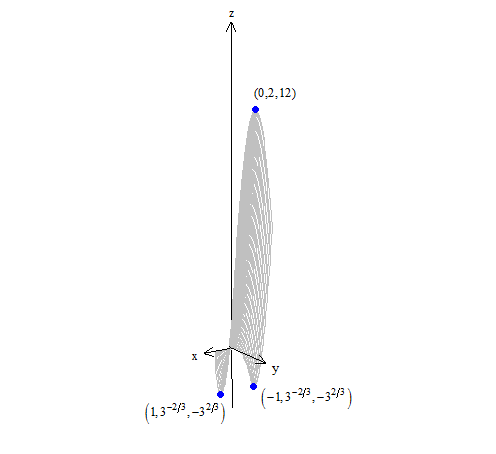Find the maximum and minimum values of f(x,y) = 5x2 − 4y2 + 3x − 2y − 1 on the triangular region D enclosed by the vertices (0,0), (1,0) and (0,1).
• The region D is closed and bounded and f is continuous on D, so there exists a maximum and minimum value on D.
•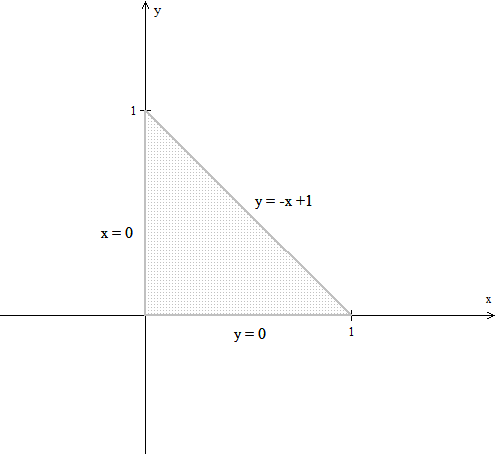• We first look for critical points on that region, [df/dx] = 10x + 3 and [df/dy] = − 8y − 2.
• So [df/dx] = 0 gives x = − [3/10] and [df/dy] = 0 gives y = − [1/4]. The critical value is f( − [3/10], − [1/4], ) but note that ( − [3/10], − [1/4], ) ∉ D.
• To check for extrema on our boundary we observe the three cases: f(0,y), f(x,0) and f(x, − x + 1).
• For f(0,y) we have z = − 4y2 − 2y − 1 which is a part of a parabola on D. The function is decreasing, hence it has possible extrema at (0,0, − 1) and (0,1, − 7).
• For f(x,0) we have z = 5x2 + 3x − 1 which is a part of a parabola on D. The function is increasing, hence it has possible extrema at (0,0, − 1) and (1,0,7).
• For f(x, − x + 1) we have z = 9x3 − 3x − 3 which is a part of a parabola on D. Note that the vertex of this parabola is at ( [1/6],[5/6], − [13/4] ) hence it is a possible extrema. Another other extrema occurs at (1,0,3).
•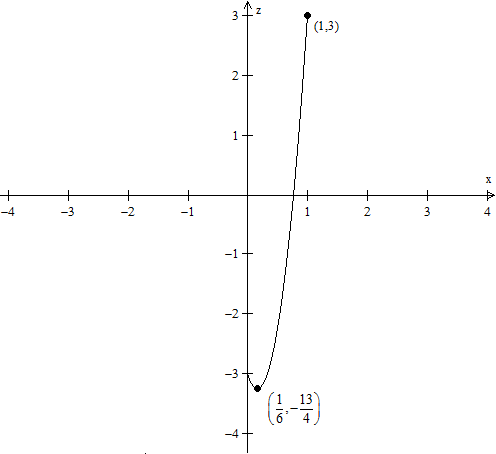Our possible extrema are then (0,0, − 1), (0,1, − 7), (1,0,7), ( [1/6],[5/6], − [13/4] ) and (1,0,3). Hence our minimum value is (0,1, − 7) while our maximum value is (1,0,7).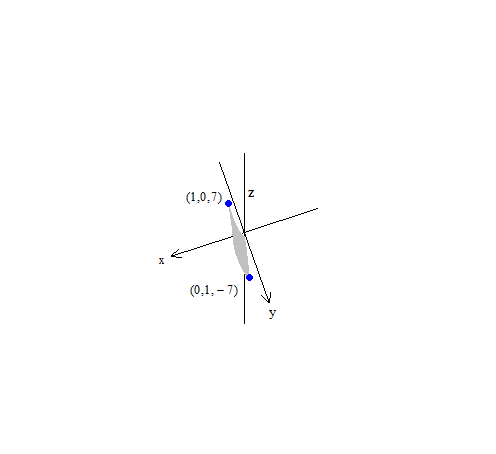Find the maximum and minimum values of f(x,y) = [(x2)/4] − [(y2)/9] on the triangular region D bounded by the parabola y = x2 and the line y = 1.
• The region D is closed and bounded and f is continuous on D, so there exists a maximum and minimum value on D.
•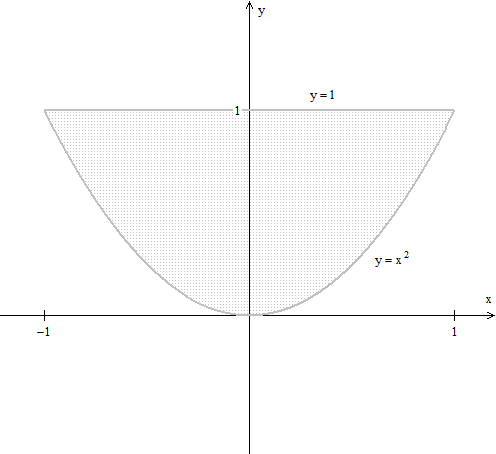• We first look for critical points on that region, [df/dx] = [x/4] and [df/dy] = − [2y/9].
• So [df/dx] = 0 gives x = 0 and [df/dy] = 0 gives y = 0. The critical value is f(0,0) = 0, a possible extrema is (0,0,0).
• To check for extrema on our boundary we observe the two cases: f(x,x2) and f(x,1).
• For f(x,x2) we have z = [(x2)/4] − [(x4)/9] which has extrema at x = ±[3/(2√2 )], but such x values are outside D.
• In the interval − 1 ≤ x < 0 the function is decreasing while on the intervals 0 > x1 the function is increasing. Aside (0,0,0) we have the possible extrema ( − 1,1,[5/36] ) and ( 1,1,[5/36] ).
•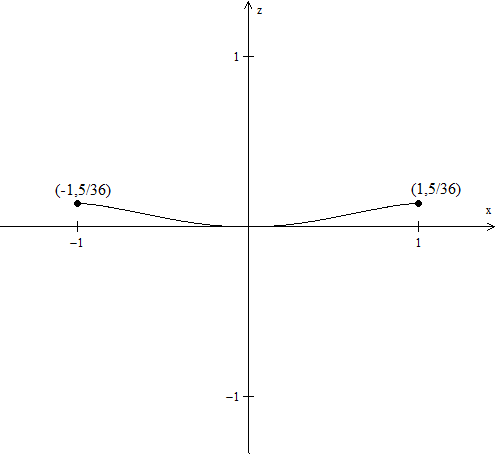• For f(x,1) we have z = [(x2)/4] − [1/9] which has an extrema at x = 0. The function is a parabola decreasing from − 1 ≤ x < 0 and increasing from 0 > x1. The only new extrema we obtain is ( 0,1, − [1/9] ).
Hence our possible extrema are (0,0,0), ( − 1,1,[5/36] ), ( 1,1,[5/36] ) and ( 0,1, − [1/9] ). Our maximum values are ( − 1,1,[5/36] ) and ( 1,1,[5/36] ) while our minimum value is ( 0,1, − [1/9] ).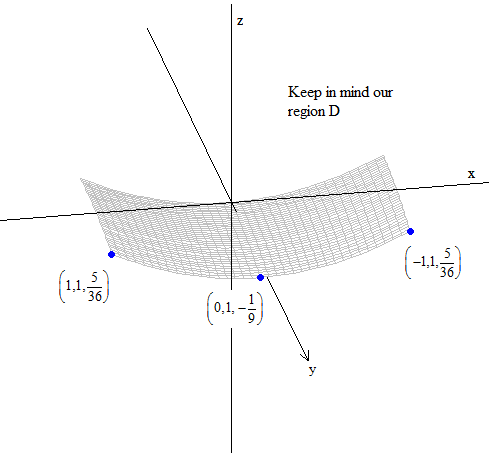Find the absolute maximum and minimum values (if any) of f(x,y) = ex + y2.
• Note that there is no guarantee that there exists a maximum or minimum value. We can begin by finding our critical points.
• For [df/dx] = ex = 0 we have no possible values for x. For [df/dy] = 2y = 0 we have y = 0. Our critical points occur at (x,0,ex).
Note that z = ex is an increasing function with no extrema. Hence f(x,y) = ex + y2 has no maximum or minimum values.
Find the absolute maximum and minimum values (if any) of f(x,y) = (x − 1)2 + 5y.
• Note that there is no guarantee that there exists a maximum or minimum value. We can begin by finding our critical points.
• For [df/dx] = 2x − 2 = 0 we have x = 1. For [df/dy] = 5 = 0 we have we have no possible values for y. Our critical points occur at (0,y,1 + 5y).
Note that z = 1 + 5y is an increasing function with no extrema. Hence f(x,y) = (x − 1)2 + 5y has no maximum or minimum values.
Find the absolute maximum and minimum values (if any) of f(x,y) = [1/(x2)] + y2.
• Note that there is no guarantee that there exists a maximum or minimum value. We can begin by finding our critical points.
• For [df/dx] = − [2/(x3)] = 0 we have x undefined at 0. For [df/dy] = 2y = 0 we have y = 0. Our critical points occur at ( x,0,[1/(x2)] ).
Note that z = [1/(x2)] is an increasing function from − ∞ ≤ x < 0 and a decreasing function from 0 > x >∞. Hence f(x,y) = [1/(x2)] + y2 has no maximum or minimum values.
Find the absolute maximum and minimum values (if any) of f(x,y) = x(x − y − 1).
• Note that there is no guarantee that there exists a maximum or minimum value. We can begin by finding our critical points.
• For [df/dx] = 2x − y − 1 = 0 and for [df/dy] = − x = 0 or x = 0. Then 2(0) − y − 1 = 0 yields y = − 1. Our critical point is at (0, − 1,0).
Note that f(1,1) = − 1 and f(1, − 2) = 2, so f(0, − 1) is neither a maximum or minimum and f(x,y) = x(x − y − 1) has no maximum or minimum values.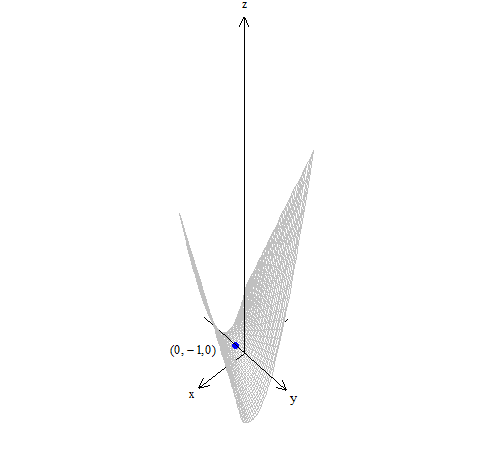Find the maximum and minimum values of f(x,y) = x2 + y2 + [1/(x2 + y2)] on the region D = { (x,y)|x2 + y2 ≤ 1}.
• The region D is closed and bounded and f is continuous on D, so there exists a maximum and minimum value on D. We first look for critical points on that region.
•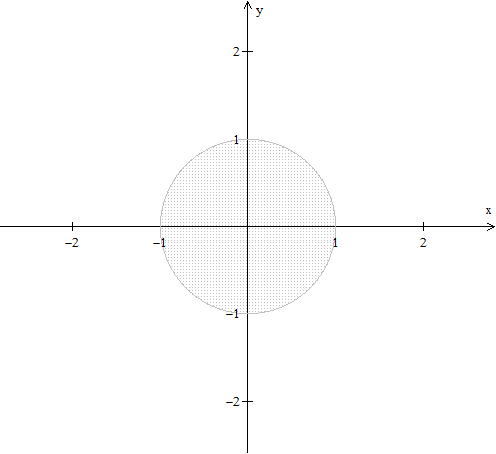• So [df/dx] = 2x − [2x/(x2 + y2)] = 0 which results in x2 + y2 = 1. Also [df/dy] = 2y − `[2y/(x2 + y2)] = 0 results in x2 + y2 = 1. Our extrema are the points satisfying x2 + y2 = 1.
• Note that these points are the boundary of D, so our extrema must occur at x2 + y2 = 1.
• We can use the parametric representation for a circle to find the extrema at the boundary. Set C(t) = (cos(t),sin(t)).
• Then f(C(t)) = ( cos(t) )2 + ( sin(t) )2 + [1/(( cos(t) )2 + ( sin(t) )2)] = 2.
• Note that no matter what value of t we input our output is always 2. Hence the points satisfying x2 + y2 = 1 are either a minimum or a maximum.
• Now, if x < 1 and y < 1 then x2 + y2< 1 but [1/(x2 + y2)] > 1. So f(x,y) = x2 + y2 + [1/(x2 + y2)] > 2 for nonboundary points (x,y) ∈ D.
It follows that the boundary points are all minima.

*These practice questions are only helpful when you work on them offline on a piece of paper and then use the solution steps function to check your answer.

### Further Examples with Extrema

Lecture Slides are screen-captured images of important points in the lecture. Students can download and print out these lecture slide images to do practice problems as well as take notes while watching the lecture.

• Intro 0:00
• Further Example with Extrema 1:02
• Example 1: Max and Min Values of f on the Square
• Example 2: Find the Extreme for f(x,y) = x² + 2y² - x
• Example 3: Max and Min Value of f(x,y) = (x²+ y²)⁻¹ in the Region (x -2)²+ y² ≤ 1

### Transcription: Further Examples with Extrema

Hello and welcome back to educator.com and to multi-variable calculus.0000

Today we are going to continue our discussion of maximum, minimum.0004

We are actually just going to do example problems. The last lesson, we finished off with the main theorem of closed and bounded sets and the existence of absolute max's and absolute min's on that particular closed and bounded set.0009

Now, we are going to just going to do some examples to get an idea of the different things that can come up when we are dealing with a particular function.0022

Really, this lesson is just about getting comfortable with all of the different tools that we have to use - all that... everything that we know, whatever it is that we can bring to solve a particular problem regarding a maximum and minimum.0030

Remembering all along that really what we are trying to find is those points where the function obtains a maximum, and obtains a minimum.0045

Ultimately this is really just a straight mathematics problem. Nothing more than that. We definitely do not want to lose the forest from the trees.0053

Okay. Let us get started. Our first example is going to be, so we will say let f(x,y) = x + y. Nice, simple function of 2 variables.0062

So, we want to find the max and min values of f on the square with coordinates + or -1, + or -1.0080

Okay, this is what this means. So, we have a square at... sorry... (1,1), (1,-1), (-1,1), (-1,-1).0118

So we have this square, and this is closed. This is definitely a closed and bounded set, so in the entire x, y plane, our domain is just what is inside here and what is on the boundary.0135

The theorem says that the function on a closed and bounded set contains a maximum and a minimum. Our job is to find that maximum and minimum, the points on there and the actual value of the function.0151

So, we have an interior and we have the boundary, so let us just go ahead and do what we do.0165

The first thing we do is, of course, take the partial derivatives, set them equal to 0. If they are equal to 0, then start with the local max's. In other words, the interior points.0170

So when I do df/dx, I get 1, and if I do df/dy, I also get 1, so as it turns out, this particular function on the entire x, y plane, there is nowhere... there is no critical point, because there is nowhere that the derivative is equal to 0.0179

What this tells me is that if there is a maximum and a minimum, which there is, it is guaranteed by the last theorem, by the last lecture, that maximum and min occur somewhere on the boundary.0203

It does not occur anywhere inside. It is going to happen somewhere on here.0214

Now, I am going to end up having to evaluate the function on the boundary. That is what is going on here.0221

Well, let us just start. So what I am going to do is I am going to call this line segment on the left, I am going to call it C1, and I am going to call this line segment here C2, and I am going to call this one C3, and I am going to call this one C4.0229

I am going to evaluate the values of the function all along all of these boundaries and see which number gives me the biggest value. That is how I do it.0245

So, let us go ahead and let us see. Let us check the boundary points.0257

Well, let us do C1 first. So C1, every single point on here has the coordinate (-1,y), okay?0271

So, f(-1,y) has a max at (-1,1), and the value of f(-1,1) is equal to 0.0285

Well, it has a min at (-1,-1) and the value at (-1,-1) equals -2. That is what I am doing.0308

I am just evaluating, now, everything on the boundary. Once I collect all of my values of all the different values of the function on the boundaries, the max's and the min's, I am just going to pick the biggest, and I am going to pick the smallest.0325

Now, let us try C2. So on here, the max was up here, and the min was here. Everything else was just in between. Again, we do not care about what was in between, we just about the max and the min, somewhere on there.0340

So, here, all of the points along this particular line have the y coordinate equal to -1, so f(x,-1). Okay.0355

So, f of all these points that have the same y coordinate, it is the x value that varies.0372

So, f(x,-1), it has a max at 1... no, wait... 1, 1... wait a minute... where I am I? let us see... 2... 1, 1, -1, oh, you know what? I actually... oops I think I made a little mistake here. Let me go ahead and reparameterize.0377

Let me go ahead and put C2 here, C3 here, and C4 here. So C2, I am actually going to be doing this line.0411

So, same thing. Instead of x,-1, it is actually going to be (x,1). So y value is 1 and x varies as we move from left to right.0421

So the maximum is going to be at (1,1), in other words, that point, and the value at (1,1) is going to be 2 and it is going to take its minimum value on this line over there.0433

So, it has a min at (-1,1), and f(-1,1), oh well when I put it in f, I get 0.0450

Let me go ahead and move on to the next page, and let me rewrite f again, so that I have it. It is going to be x + y, and I am also going to redraw my square real quickly, just because I would like to know what it is that I am looking at.0468

So I think we had C1, C2, C3, and C4. Okay. So now let us do C3. Now we are going to check the values along C3.0489

The coordinate of C3, the x value is going to be fixed at 1, and it is the y value that is going to vary.0500

So here, it is going to be (1,y), something like that. So f(1,y), well it has a max at (1,1) and f(1,1) is 2.0509

It has a min at (1,-1), which is this point and the value is 0. I am just doing this systematically. Just go through every single... cover the entire boundary, just make sure that all of the points are covered.0530

Now we will do C4, which is write down there, and the coordinates for C4, well the y value is fixed at -1, and it looks like the x value that is actually going to vary.0553

f(x,-1), that has a max at... it looks like that is the point at (1,-1), and the value of (1,-1) is equal to 0.0565

Then, it has a min at (-1,-1), which is that point. The value of (-1,-1) is equal to 2, or -2... sorry about that.0593

So, on the closed and bounded region, let us just call this s on that square, so on the closed and bounded region s, the maximum of f if I look through all of these values, the maximum is going to be 2, and the min is going to be -2. That is it.0614

Okay. Let us do another example here. Let us actually go do a different page. Example 2.0644

This time we want to find the extrema for f(x,y) = x2 + 2y2 - x.0656

Let us see what is going to happen here. Well, let us go ahead and start by doing what we always do. Now notice that in this particular case they just said find the extrema of the function f(x,y) = x2 + 2y2 - x.0680

They did not specify a domain, they did not actually specify some region. They did not say whether it was open or closed. When they do not do that, they are just saying over the entire x, y plane.0694

That is it. When they do not specify a region, your assumption is just that it is over the entire plane.0705

So, in this particular, we are going to be looking for absolute max, but it is actually going to end up being a local max, or an absolute min, or a local min, because we do not have a boundary that is keeping us contained.0710

Any max or min that takes place is going to end up being some critical point. That is what is going on here.0726

So, let us do what we always do. Let us go ahead and find the... so we will do df/dx, so the partial derivative with respect to x, it looks like it is going to be 2x-1.0732

The partial derivative with respect to y... it looks like it is going to be 4y.0744

So, we set each of those equal to 0, so we end up with y = 0 as the y point, and 2x-1, x is going to equal 1/2. There you go.0753

Our critical value... so let us write, our critical value equals the point (1/2,0). That (1/2,0), the derivative = 0. It is a critical point.0765

Now we just need to find out if it is a max, or if it is a min. So, here is where you are have to sort of stop and make some decisions about how you are going to proceed.0779

Are you going to do the same thing that you did before? Where you take the point (1/2,0), also it is going to be some like that and we can do what we did in one of the examples from the previous lesson.0792

We can hold 1 variable fixed and move a little bit to the left, move a little bit to the right and hold y fixed, or we can hold x fixed and we will move a little bit up and a little bit down, and we will check the value of the function around that critical point to see how the function behaves.0802

To see if all of the values around it are bigger than what the value of the function is there, or less than what the value of the function is there. To see if it is actually a maximum or minimum.0821

First of all, let us go ahead and find out what the value of the function is at (1/2,0).0833

So f(1/2,0) is going to equal... 1/22 is 1/4, let us see... 0, this is 0... -1/2 equals -1/4, so the value of the function at the critical point is -1/4.0839

Here is where you stop and think about what you are going to do. Are you going to go through this process? Which you can, it is not a problem, you will get the answer. It is perfectly acceptable to do that.0859

But in this case, let us look at the function. We know the value is -1/4.0870

Well, take a look at the first two terms of this function. You have got x2 and you have got y2.0876

So, in this particular case, both of these terms, no matter what value of x you put in, no matter what value of y you put in, you are always going to end up with positive terms here.0884

Because they are positive terms, you are just subtracting this final x, you are always going to end up getting some number. In other words f(x) is always going to be bigger than f(1/2,0).0898

So these are positive. That is what is going on here. Just sort of by looking at this function and stopping and thinking about it, here is what you can do.0911

So, always positive, so... because this and this are squared terms... let me write it again actually.0921

So, f(x,y) = x2 + 2y2 - x. This is always going to be a positive term. This is always positive. Therefore, f(x,y) is always going to be > or = to 1/4, which is the value of the function there.0931

So no matter if I move this way, this way, this way, this way, no matter which way I move, because these are both squared terms and they are both positive and it is always going to be a value that is bigger than -1/4.0963

Therefore, this is actually a minimum. In other words, all of the values around the function are going to end up being bigger... so it is going to be something like this. It is going to go that way.0975

If I hold the other variable fixed, it is going to go that way. So this is a minimum.0988

So, min at (1/2,0) = -1/4, so this is where the minimum takes place. The value of the minimum is -1/4, so that is it.0995

Again, you are welcome to go ahead and go through the process that we did of holding a variable fixed, moving a little this way, moving a little this way, you are going to get the same answer.1013

In this case it was nice where we had a particular function, look at it, stop and think for a second, and say, Okay, well both of those terms are positive therefore at that value it is always going to be -- the value of the function is always going to be -- bigger than -1/4.1021

That is what is happening. Let us do another example. Let us see, example 3.1038

Find the max and min of f(x,y) = (x2 + y2)-1.1055

Okay, so slightly more complicated function here. In the region -- we are going to add one more bit of complication here -- in the region x-22 + y2 is less than or equal to 1.1075

Now I have not only specified a function, I have specified a region except now the region is given as an equation, so this is an equation of a circle that is centered at (2,0).1096

Let us go ahead and draw this out because we can. Any time you can actually draw a picture is is a good idea. So this is 1, this is 2, so this is the center of the circle. There is that, there is that, and there is 3.1107

So, we have a circle and it is less than or equal to 1 of radius 1, okay? It is closed, this is a closed and bounded region.1122

What we are saying here is on this region, all the interior points including the boundary points, what is the maximum value that this function obtains, and what is the minimum value that this function obtains. That is all that we are doing here.1133

Well, let us just start and see where we go. So, let us just take the df/dx.1148

When we take the first partial derivative, this is going to equal -x2 + y2 × the derivative of what is inside, which is 2x.1154

df/dy = -x2 + y2 × 2y. Again, we are just taking the derivative of this function, this is a composite function, so we take -1 × this... -2 power, sorry about that, and then of course we take the derivative of what is inside.1171

Since we are differentiating with respect to y, it is just 2y here. The first one we differentiated with respect to x.1194

Not notice, take a look at this. Well, for one thing, the function is not even defined at (0,0). You cannot even have that because this is... okay, let me rewrite this, so this is going to be (-2x/x2 + y2)2,1202

This is going to be -2y/(x2 + y2)2.1220

First of all, this function is not even defined at (0,0), however for every other value of x and y, the derivative never equals 0.1229

So, df/dx does not equal 0 anywhere on the domain, anywhere in the region.1240

Not that we were concerned with (0,0) because (0,0) is not even part of this. But anywhere on this region, the derivative does not equal 0, and neither does df/dy.1250

df/dy does not equal 0 anywhere on the region in question, because the derivative does not equal 0 anywhere on that region, we know that it is not going to take place anywhere on the interior.1260

So, the max and min of this function is going to take place on the boundary. It is the boundary that we need to concern ourselves with.1276

Here is where it starts to get kind of interesting. So, let us go through this very, very carefully.1282

Now, let me go ahead and move on to another page. I am actually going to redraw it so that I have it available for me.1290

I have this 1, 2, 3, just do a quick drawing... so, I have that. The unit circle centered at 2.1298

So, the boundary is x - 22 + y2 = 1. That is the boundary. The region was < or = to 1, but the boundary is at 1.1308

How can we find the values of the function -- let me rewrite the function -- so, find values of f(x,y), which is equal to x2 + y2... let me write it actually as a fraction so that we have positive exponents.1328

1/(x2 + y2)1. So that is actually what we are looking... how can we find the values of this function on this circle?1356

The value of this function on this circle. This is a composite function. I need to be able to take this, I need to be able to form f, so this is some... I need to be able to form f(g). This is g, this is f. I need to form the composite function.1370

Here is what I am going to do. I am going to go ahead and parameterize this circle, and express it as a function of one variable, t.1390

Let us go ahead and parameterize this circle. Well, I know what the parameterization of a circle is in general.1397

So, a general circle, the parameterization is just c(t) = cos(t)sin(t), right? That is the parameterization of a unit circle centered at the origin. But this one is not centered at the origin, it is centered at 2.1403

My parameterization for this function here, x - 22 + y2 = 1, looks like this. Equals 2 + cos(t)sin(t).1420

If you were to put 0, pi/2, 3pi/2, and 2pi into t, you will get this point, this point, this point, and this point. That is your parameterization moving in that direction.1434

This is a parameterization of this function. All I have done is taken an implicit function, and I have expressed it parametrically.1448

Now I can actually form f(c(t)). That is what is actually going on here. So, c(t) is this, this is f, I am going to form f(c(t)).1458

f(c(t)) is equal to, well, it is going to be 1/x2, well x is this. That is x, that is y, so it is going to be 2 + cos(t)2 + sin(t)2.1470

Now we are going to go ahead and actually multiply everything out. It is going to equal 1 over, well, 2 × 2 is 4, 2cos(t)2cos(t) is + 4cos(t), + cos2(t) + sin2(t). That is the denominator.1500

Well, cos2(t) + sin2(t) is the pythagorean identity. That is equal to 1, so 4 + 1.1523

So my f(c(t)) = 1/5 + 4cos(t).1530

There we go. I found a way of expressing my function as a single variable, a function of t, this is my function. This is f(x,y).1546

Now, all I need to do is find the values of t that make this a maximum, and find the values of t that make this a minimum.1557

Well, this is actually really, really simple here. Notice this cos(t).1565

Let us just... well, let us just stop here. Let us just say f is a max, the function chooses its maximum value when the denominator is the smallest and f achieves this number here.1572

Achieves a minimum value, a smallest value, when the denominator is the biggest, right?1600

This is the function, big denominator, small value. Small denominator, big value. That is what is going on here.1615

Now let us go ahead and find what this is. Well, what do we know about cos(t)?1625

Well, cos(t) has its biggest value of 1, and its smallest value at -1. It ranges between -1 and 1.1629

Therefore, it is going to achieve... so cos(t) = 1, when t=0... so we want this value here. We want to achieve the biggest and smallest value. Well, we want to achieve 1, and we want to achieve -1.1640

Okay. It is equal to 1 when we have cos(t) = 0, therefore when t = 0, that means the cos(0) is 1. That means 1 + 5 × 4 × 1. It is going to be 1/9.1666

Therefore at t=0, that is when it is going to achieve its minimum value.1693

Now the minimum value of cos(t)... cos(t) achieves -1 when it is equal to pi. So when t = pi, the cos is equal to -1.1699

Well -1 × 4 is -4, 5 -4 is 1, so now it achieves its maximum value there. So let us actually just write this out.1711

When t = 0, c(t) = 2 + cos(0)sin(0) = (3,0). That is the point at which it is going to achieve one of its extrema value.1728

Well, f(c(t)) is going to equal 1/5 + 4, which equals 1/9. That is the minimum.1752

Now, when t = pi, cos(t) = -1. c(t) = 2 + cos(pi) × sin(pi), which is equal to (1,0).1768

When I put (1,0) into that function, f(c(t)) = 1/1, because remember, we had 1/5 + 4 × cos(t). 5 + 4 × cos(t).1795

When t = 0, it is 1/9, so it is 1/9. The value of the function at the point (3,0) is equal to 1/9.1821

When t = pi, that is when it is at its smallest. The smallest value of cos is when t = pi.1833

When t = pi, well cos(pi) is -1, 4 × -1 is -4, 5 - 4 is 1. So the value of the function is 1.1840

At the point (1,0), the value of the function is 1. That is the maximum.1851

At the point (3,0), the value of the function is 1/9. That is the minimum.1859

Here is what we have done. Here is our circle. 1, 2, 3, we have a circle here. That looks like an ellipse, so let us make it look a little bit like a circle.1863

We have this circle, so at the point (1,0), here is where it achieves its maximum value of the function. At the point (3,0), here is where the function actually achieves its minimum value.1873

We did this by just taking our function, parameterizing it, putting it into our region, parameterizing the curve, putting that parameterization, forming (f(c(t)) like we have been doing for a while, and then we just found the values of t that make the function a maximum or a minimum.1886

The only real problem here is keeping track of all of the mathematics. There is a lot on the page.1909

There are a lot of numbers floating around, there is a lot of points floating around. The only thing that you have to do is make sure that you differentiate between the point at which the maximum or minimum is achieved.1914

In other words, these points. Those are the points at which they are achieved, and the actual value of the function at those points, that is here, and that is here.1925

Again, we want to rely on the techniques that we have at our disposal, but really what we want to rely on is our intuition and what we know about functions and how they behave and how numbers work.1935

When you get to this point, you can reason out the rest. When this is the biggest, the number is going to be the smallest. When this is the smallest, this number is going ot be the biggest.1947

You just need to go step by step and make sure that everything is there. Okay. I hope that this has worked out and everything is well.1958

Thank you for joining us here at educator.com and multivariable calculus. We will see you next time.1963

OR

### Start Learning Now

Our free lessons will get you started (Adobe Flash® required).